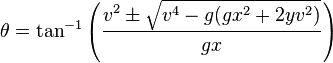# Angle for a projectile that must clear 2 walls

## Homework Statement

A cannon is aimed at a target located 72m away from the cannon and 20m above the ground. The cannon ball, when fired, will have the initial speed of 32m/s, but it has to clear a 13m wall between the cannon and the target as, shown. At what angle should the cannon be fired?

**Diagram attached

## Homework Equations

Dy=(Voy)(t)+(1/2)(a)(t)2

Vx=Dx/t

## The Attempt at a Solution

I have tried to do this question many different ways, but I have not been able to find the correct answer.

Here is what I have tried:
For the first wall Dx= 15, Dy=13, Vx=32cos($$\theta$$) Voy=32sin($$\theta$$)
and since Vx=Dx/t ,Dx/Vx=t then t=15/32cos($$\theta$$)
Dy=Vot+1/2(a)(t)2

13=(32sin($$\theta$$)(15/32cos($$\theta$$)+(1/2)(-9.8)(15/32cos(theta)2

I am going to stop here for help because I don't think I am doing this correctly.

Thank you! =)

#### Attachments

•Untitled.png
15.1 KB · Views: 361

## Answers and Replies

SammyS
Staff Emeritus
Science Advisor
Homework Helper
Gold Member
Try to hit the target first. You will likely get two values of θ that will allow the projectile to hit the target. See if either or both or neither will allow the projectile to clear the wall.

Therefore, use Dx = 72 m and Dy = 20 m

Should I use this equation: Dy=(Voy)(t)+(1/2)(a)(t)^2 and substitute 72/32cos(theta) in for t?

thanks

SammyS
Staff Emeritus
Science Advisor
Homework Helper
Gold Member
Should I use this equation: Dy=(Voy)(t)+(1/2)(a)(t)^2 and substitute 72/[32cos(theta)] in for t?

thanks

Yes. Also, Dy = 20 , V0y = 32 sin(θ) .

I'm having some problems with the algebra in the equations; I haven't done much work with trig identities.

Here's my attempt at solving the equaiton:

Dy=20, Dx=72, Voy=32[sin$$\theta$$], and like we established: t=72/32[cos$$\theta$$]
Dy=Voyt + 1/2(a)(t)2

20= 32[sin$$\theta$$] (72/32[cos$$\theta$$]) + 1/2(-9.8){(72/32[cos$$\theta$$]}2

Here is where I get mixed up:

{72/32[cos$$\theta$$]}2

Would this be equal to 722/322[cos$$\theta$$]
or 722/322cos2$$\theta$$
or 722/322 [cos(theta)]2

or even 722/32[cos(theta)]2?

I was searching on the internet for help on this question, and I came across a formula that was from wikipedia's "Trajectory of a projectile page"

It says you can use the following formula to find the Angle θ required to hit coordinate (x,y)and it worked!
I got 63 degrees and 42 degrees, just like to book says. Thank God my instructor said that we can copy any formulas that are useful onto the formula sheet!

What do you think of this? Is there any easier ways to do this?

Thanks!

SammyS
Staff Emeritus
Science Advisor
Homework Helper
Gold Member
I'm having some problems with the algebra in the equations; I haven't done much work with trig identities.

Here's my attempt at solving the equation:

Dy=20, Dx=72, Voy=32[sin$$\theta$$], and like we established: t=72/32[cos$$\theta$$] When you write it this way, cos(θ) is not in the denominator, but it should be.
Write: t=72/[32cos(θ)]

Dy=Voyt + 1/2(a)(t)2

20= 32[sin$$\theta$$] (72/[32cos$$\theta$$]) + 1/2(-9.8){(72/[32cos$$\theta$$]}2

Here is where I get mixed up:

{72/32[cos$$\theta$$]}2

Would this be equal to 722/322[cos$$\theta$$] No
or 722/322cos2$$\theta$$ Yes, for the expression you asked about.
or 722/322 [cos(theta)]2 Yes, for the expression you asked about.

or even 722/32[cos(theta)]2? No

I was searching on the internet for help on this question, and I came across a formula that was from Wikipedia's "Trajectory of a projectile page"

It says you can use the following formula to find the Angle θ required to hit coordinate (x,y)and it worked!
I got 63 degrees and 42 degrees, just like to book says. Thank God my instructor said that we can copy any formulas that are useful onto the formula sheet!

What do you think of this? Is there any easier ways to do this?

Thanks!
Yes, the algebra is quite messy. The Wikipedia article you mentioned does show how the formula is obtained. IMO, knowing where it comes from is much more important than using some formula you don't understand.

20 = 32[sinθ] (72/[32cosθ]) + 1/2(-9.8){(72/[32cosθ]}2

→ 0 = 1/2(9.8){(72/[32cosθ]}2 - 32[sinθ] (72/[32cosθ]) + 20

→ 0 = (4.9)722sec2θ/322 - 72tanθ + 20

→ 0 = (4.9)722(1+tan2θ)/322 - 72tanθ + 20

→ 0 = (4.9)722tan2θ/322 - 72tanθ + 20 + (4.9)722/322

Use the quadratic formula to solve for tanθ .